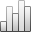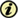Acta Stereologica

0351-580XMore Statisticssince 05 December 2013 :
View(s): 138 (4 ULiège)
Leszek Wojnar

### On some misunderstanding of fracture roughness parameters

Article#### Abstract

A corrected equation describing the upper bound for RL—RS relation was derived as Rs = (π/2).RL – (π2 – 8)/4.

Due to some misunderstanding the above equation was called incorrect. The nature of this misunderstanding is explained and an experimental proof of correctness is presented.

Keywords : fractography, roughness parameters, stereometric equations, surface rougness